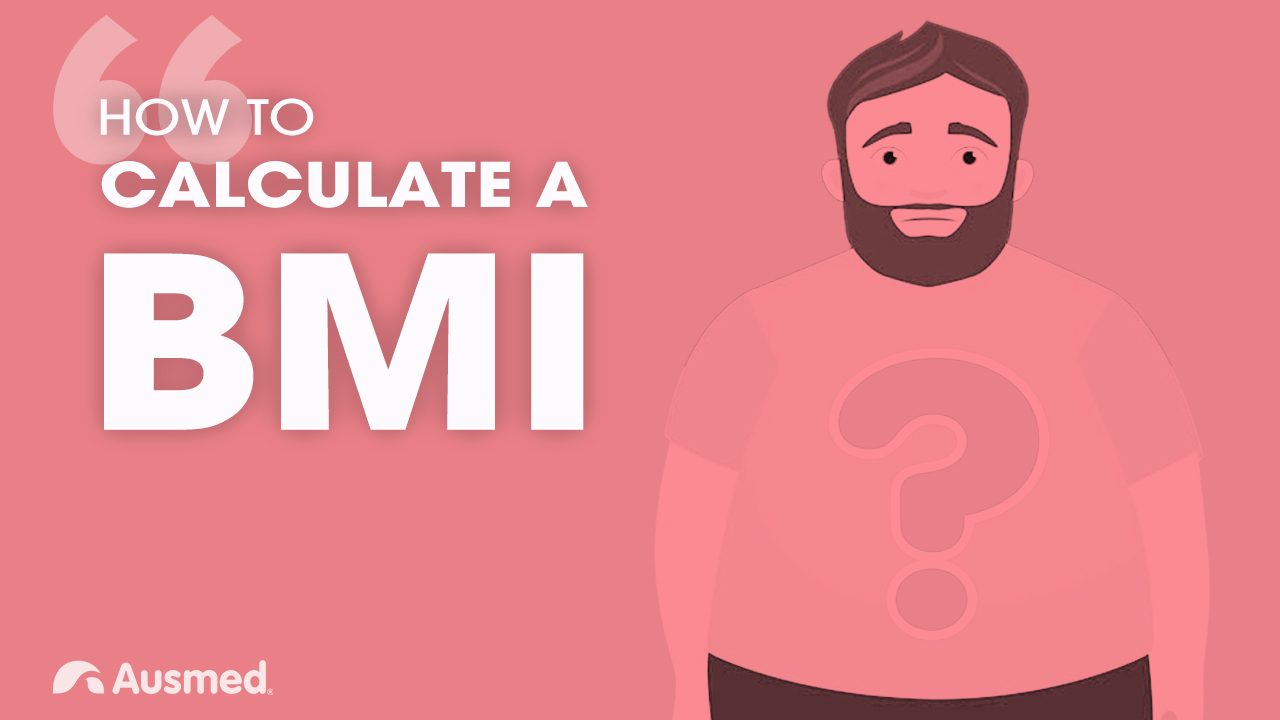# How to Calculate a Body Mass Index (BMI)

CPD

## 1m### Ausmed Explains... How to Calculate a Body Mass Index (BMI)

Body mass index (BMI) is a measurement used to classify weight.

It is calculated as the ratio of weight in kilograms (kg) divided by height in metres-squared (m2), i.e.:

BMI = WEIGHT (kg) / HEIGHT (m2).

How about calculating your own BMI? Find out what it means by comparing your results to the table in the video.

## Learner Reviews

3.6

5 Total Rating(s)##### Anne Podmore
01 Jul 2019

Very clear and concise, Good to have as a reference.##### Michelle mckillop
01 Jul 2019

good basic overview of BMI calculation and interpretation##### Clare Bock
15 Jun 2019

A basic 1 min refresher of a nursing assessment skill